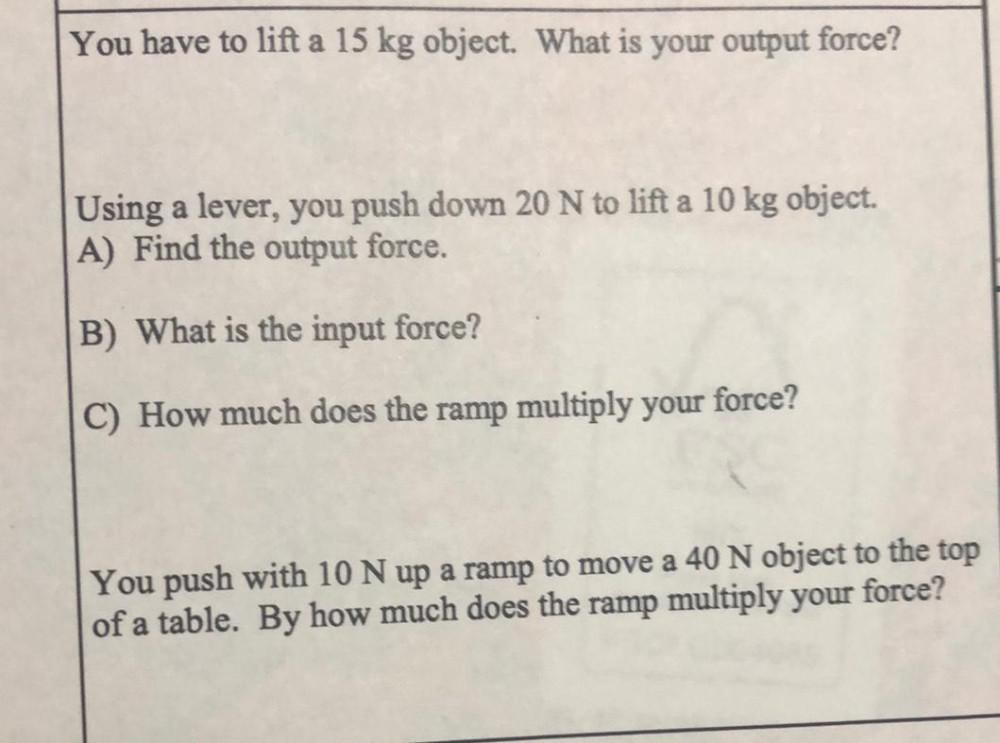Question:

# You have to lift a 15 kg object. What is your output force? Using a lever, you push down 20 N to lift a 10 kg object. A) Find the output force. B) What is the input force? C) How much does the ramp muYou have to lift a 15 kg object. What is your output force? Using a lever, you push down 20 N to lift a 10 kg object. A) Find the output force. B) What is the input force? C) How much does the ramp multiply your force? You push with 10 N up a ramp to move a 40 N object to the top of a table. By how much does the ramp multiply your force?